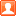# 江城探景（20）之一———徒步行走在武昌江滩上发表于 3 天前 | 显示全部楼层 |阅读模式
 [size=0.4]江城探景（20）之一———徒步行走在武昌江滩上 [size=0.36] [size=0.36]楚风雅颂[size=0.36] [size=0.36]我生在长江边，长在长江边，曾经在长江的滚滚波浪中畅游，看那白帆，看那渔船，看那航标灯，看那飞翔的白鸥，看那欢快戏水的江豚……。[size=0.36]        远逝的青春岁月，仿佛映画在长江的激流浪花之中，如今畅想东逝水，满怀豪情忆往昔。[size=0.36][size=0.36]　　把平凡的平淡生活，过成心里的美丽和愉悦，那不是梦。[size=0.36]        徒步游览武昌江滩，是很早之前的一个惦念，也是一个梦，今天决定将此变为现实。[size=0.36][size=0.36]　　充满阳光的早晨，乘坐熟悉的566公交车，从东亭站直接奔驶张之洞路花堤街，车程一小时。[size=0.36][size=0.36]　　武昌江滩西南起于武船，东北止于二七长江大桥下的武昌区与青山区堤防的分界线。[size=0.36]      从西到东经过紫阳路、平湖门、大成路、武汉长江大桥桥头堡、汉阳门、中华路、中山路、积玉桥、新生路、四马路、秦园路……。[size=0.36]        武汉音乐学院与张之洞路花堤街之间，堤边是一个拐弯处，此地便是武船的区域，武昌区江滩的起始点。[size=0.4]起点：武  船[size=0.36][size=0.36]　　登上长江堤边，横跨长江两岸的鹦鹉洲长江大桥，映入眼帘。[size=0.36][size=0.36]　　武汉音乐学院大门，公交车站，还有一旁格外招人喜欢的雕塑。[size=0.36][size=0.36]　　眼见长江进入了夏季的汛期，江水已淹没了江滩上的观赏亭。[size=0.4]途经：紫阳路[size=0.36][size=0.36]　　防汛的堤坝上，挂满了郁郁葱葱的翠绿。[size=0.4]路过：平湖门[size=0.36][size=0.36]　　江面上，停泊的轮船和远处的长江大桥。[size=0.4]回望：大成路[size=0.36][size=0.36]　　江滩长廊上，一位老者正在耍花刀。[size=0.4]驻足：桥头堡[size=0.36][size=0.36]　　漫步在武汉长江大桥下，穿梭于跳舞的，打拳的，唱歌的人群中，身临其境，感受热闹非凡的氛围。[size=0.36][size=0.36]　　武汉长江大桥，屹立在龟蛇两山之间，已近65年，它依然风采依旧。[size=0.36][size=0.36]　　站在武昌桥头边，眺望拔地而起的高楼大厦，无限感叹，武汉的两江四岸，发生了翻天覆地的变化。[size=0.4]漫步：汉阳门[size=0.36][size=0.36]　　漫步汉阳门，耳畔回响起冯翔的那首脍炙人口的汉味歌曲《汉阳门花园》。[size=0.36]小时候的民主路[size=0.36]﻿冇得那多人[size=0.36]外地人为了看大桥[size=0.36]才来到汉阳门[size=0.36]汉阳门的轮渡[size=0.36]可以坐船去汉口[size=0.36]汉阳门的花园[size=0.36]属于我们这些住家的人[size=0.36]冬天腊梅花[size=0.36]夏天石榴花[size=0.36]晴天都是人[size=0.36]雨天都是伢[size=0.36]……[size=0.36][size=0.36]　　缓步穿过“渡江文化长廊”的那一刻，蓦然间，想起了毛主席畅游长江的情景，想起了毛主席乘坐的“7.16”快艇，想起了武汉每年举办的“7.16”横渡长江纪念活动！[size=0.4]指点：中华路[size=0.36][size=0.36]　　武汉是一座“因码头而建，又因码头而兴”的城市，码头文化成了武汉的名片。[size=0.36]        中华路上，闻名遐迩的当属中华路1号码头。去汉口，大多数人在此乘座轮渡。[size=0.36][size=0.36]　　绕过中华路码头，登上江堤，继续前行。[size=0.4]眺望：中山路[size=0.36][size=0.36]　　在中山路上，聚集着许多令人回忆的地方。[size=0.36]        毗邻武昌江滩的常阳丽江城小区。[size=0.36][size=0.36]　　武昌红港游船码头，游客在参观了红港之后，在此乘坐夜游船，可以观欣武汉长江两岸的灯光秀。[size=0.36][size=0.36]　　武昌江堤对面的红巷、政务中心和军粮供应站。[size=0.36][size=0.36]　　站在此处的江堤上，遥望“红巷”一一都府堤，农讲所，毛泽东旧居，中共五大会址，曾经有多少革命的先辈们也是站在这里，指点江山，激扬文字：把酒酹滔滔，心潮逐浪高！[size=0.4]散步：积玉桥[size=0.36][size=0.36]　　积玉桥的“武昌江阅”和大堤口广场[size=0.36][size=0.36]江滩上的绿道标识[size=0.36]　　每一张标识，都在丈量着前行的脚步。[size=0.36][size=0.36]　　武汉人习惯指路的方向，是上下，而不是东南西北。这就是按长江的流向而确定的一一上游和下游。[size=0.36]        现在的我，是沿着下游的方向，朝武汉二桥漫步前行。[size=0.36][size=0.36]　　游武汉的江滩，汉口和武昌最大的区别就在于，逛汉口江滩重在休闲，而逛武昌江滩意在观景。[size=0.36][size=0.36]　　水天一色 长江揽胜[size=0.36]        蓝天白云下的长江岸边，林林总总拔地而起的高楼大厦，是多么的蔚为壮观。[size=0.36][size=0.36]　　一份闲暇，是在武昌江滩留下美好时光。一份快乐，是在武昌江滩寻觅美好岁月。[size=0.36][size=0.36]云楼九州长廊[size=0.36][size=0.36]逝水清波 浪下三吴[size=0.36][size=0.36]长江观景第一台[size=0.36][size=0.36]        武昌江滩新的人文景观，从这里开始，被打造的焕然一新。[size=0.36][size=0.36]天蓝江阔    心旷神怡[size=0.36][size=0.36]　　这个存留下来的标志性建筑，是武汉纺织业的历史标记。武汉人习惯叫的“一棉、二棉至六棉”，已经是老一辈武汉人心中抺不掉的印记。[size=0.36][size=0.36]　　民国武昌第一纱厂旧址石碑[size=0.36]        也就在这一地段，历史上有几家纺织厂，“五棉和六棉”还有“天一印染厂”，都建于此。而如今，都成了高档的商务小区。[size=0.36]　《长江诗廊》卧式碑　       《长江诗廊》卧式碑位于长江第一观景平台右侧堤岸坡道上，碑体采用高级花岗石整石安装，碑体表面雕刻文字与图案，阴刻与阳刻手法穿插结合。咫尺之外就是水天—色的长江美景，对应左岸林立的现代高楼，一起构成长江右岸新的文化景观。[size=0.36]《先秦诗人》圆雕         《先秦诗人》圆雕位于长江第一观景平台右侧平台植物园内，游园行人步道旁。               圆雕使用青铜铸造工艺，表面青铜绿自然氧化成色。雕塑背后两侧分别矗立竹简造型石牌，石牌表面雕刻三首诗词节选书法。        该圆雕为“直线式”半抽象雕塑，通体高4.2米，雄伟壮丽，人物气宇轩昂，与山体浑然一体，表现先秦诗人傲骨嶙峋的写意形象。寓意长江诗歌开端、傲然于世岿然不动，展现了先秦诗性的人物风采。[size=0.36][size=0.36]　　长江边，柳树下，一群游泳老者，可谓是老当益壮，勇搏激浪。[size=0.36]《两汉-诗人》人物群雕        《两汉诗人》人物群雕位于长江第一观景平台右侧平台植物园内,游园行人步道旁边。该群雕为“折线式”写实人物雕塑，由3个人物圆雕组成,使用青铜铸造工艺,表面处理成深紫色。其中站立人物高2.2米，坐式人物高约1.5米。      雕塑两侧分别放置石头造型青铜铸造件,石头表面分别铸造三首两汉时期抒发长江情怀诗词节选书法。人物与景石搭配，形成场景化人物组雕。[size=0.36]　　《两汉诗人》浑厚端庄,稳重质朴,人物或端坐冥思或信步提笔,动静各态,张弛有度，表现两汉诗人的写意形象，展现中正沉稳、宁折不弯、端庄儒雅的儒家风范。[size=0.36][size=0.36]给自己来一个自拍[size=0.36][size=0.36]　　继续前行，约2公里长的一段防洪墙，被打造成长江诗廊的核心景观：《诗词长卷·长江万里》瓷板画和《诗词长卷·中华诗人》浮雕。[size=0.36]        “故人西辞黄鹤楼”“滚滚长江东逝水”……熟悉的诗词都能在这里找到，身侧就是大江滔滔，让人不禁且行且吟。[size=0.36][size=0.36]　　走在绿荫的树林里，踏上一块块石板路，容易使人心静，心静，就会使人心中涌出许多的思念和感悟。[size=0.36]　　诗廊共有8处艺术景观点，以时间为轴线设置，分别为《长江诗廊》卧式碑、《先秦-诗人》圆雕、《两汉-诗人》人物群雕、《魏晋-诗人》人物群雕、《诗词长卷·长江万里》瓷板画、《诗词长卷·中华诗人》浮雕、《破·立》雕塑、《长江诗廊》立式碑。[size=0.36][size=0.36]　　走着走着，就有点热了。走着走着，也感觉有点累了。坐下来，喝口水，翻看拍的照片。[size=0.36][size=0.36]　　坐在江滩边，正准备发朋友圈的我，忽然听到一个熟悉的声音喊我的名字，抬头一看，原来是几十年的老朋友，竟然在此奇遇。[size=0.36]        正是应验了那句古话：一叶浮萍归大海，人生何处不相逢。[size=0.36]巧遇长江诗画旁，[size=0.36]人生有缘情义长；[size=0.36]眺望滚滚长江水，[size=0.36]相互问候暖心房。[size=0.36][size=0.36]　　“诗廊收录了由古至今描写长江意境的99首诗词，分别为先秦两汉6首、魏晋南北朝隋12首、唐五代30首、宋元29首、明清20首、当代2首。”[size=0.36]        诗廊通过圆雕、浮雕、壁画等多样的艺术表现方式，展现出诗人的典型形象及诗词的意境。[size=0.36][size=0.36]　　再往前走到月亮湾码头电厂闸口草坪，两组不锈钢制的雕塑为长江诗廊画上一个颇具现代感的句号。雕塑主体四个面以四种书法字体铸造“长江诗廊”四个大字。[size=0.36]        此处立式碑呼应起点处的卧式碑，两个铭文碑体，两种材质和形式，一个传统质朴，一个现代华丽。滚滚长江水，由古看到今。[size=0.36][size=0.36]　　长江诗廊全景式传播长江诗词文化，赋美学、有寓意、成景致，成为武汉江滩历史文化景观新亮点。[size=0.36]《破•立》雕塑         《破•立》雕塑位于月亮湾码头电厂闸口长江诗词廊结尾处草坪内，游园行人步道旁边。      《破•立》寓意“破土而出，自立强国”，由上下两部分组成。上部分高5米，为不锈钢锻造，取材幼笋造型，新生柔弱;下部分高约1.3米，为花岗岩雕刻，象征凌厉危险的环境，表面雕刻现代诗词节选书法。                《破•立》形式一虚一实，纵横有度，质感分明，极具视觉张力和艺术表现力。[size=0.36]　　长江虽然进入了禁渔期，但喜爱垂钓的人们，在武昌的江滩边比比皆是。[size=0.36][size=0.36]　　相比江对岸大气繁华的汉口江滩，武昌江滩充满了朴实的本地生活气息。[size=0.36][size=0.36]　　在夏日的夜晚，很多市民来这里吹吹江风，看看对面汉口江滩的夜景，或者放风筝、抽陀螺和锻炼身体。这里还有许多轮渡，可以坐船直达汉口。[size=0.36][size=0.36]长江啊长江[size=0.36]心爱的江[size=0.36]太阳映红你的胸膛[size=0.36]东风为你热情梳妆[size=0.36]千座青山把你拥抱[size=0.36]为你披上锦绣衣裳[size=0.36]你和我们伟大的祖国一样[size=0.36]年轻美丽[size=0.36]神采飞扬[size=0.36]长江啊长江[size=0.36]心爱的江[size=0.36]长江啊长江[size=0.36]心爱的江[size=0.36]大江两岸万千气象[size=0.36]人民豪迈勤劳顽强[size=0.36]看那满江上的白帆[size=0.36]川流不息奔向远方[size=0.36]你和我们伟大的朝代一样[size=0.36]滚滚向前[size=0.36]充满希望希望[size=0.36][size=0.36]　　武昌江滩的沿岸，许多的码头消失了，许多的沙滩消失了，还有许多水路联运的交通闸口消失了。随之所替代的，是众多高耸云端的楼群。[size=0.36][size=0.36]视频（1）武汉长江大桥 [size=0.26]00:40 [size=0.36][size=0.36]视频（2）桥头堡下 [size=0.26]01:24 [size=0.36][size=0.36]视频（3）横渡长江诗廊 [size=0.26]00:39 [size=0.36][size=0.36]视频（4）武汉三镇 [size=0.26]00:33 [size=0.36][size=0.36]视频（5）长江堤上 [size=0.26]00:26 [size=0.36][size=0.36]视频（6）江滩晨练 [size=0.26]00:45 [size=0.36][size=0.36]视频（7）长江美如画 [size=0.26]00:34 [size=0.36][size=0.36]视频（8）长江诗篇 [size=0.26]01:49 [size=0.36][size=0.36]视频（9）长江诗画 [size=0.26]01:09 [size=0.36][size=0.36]视频（10）岸边的欢笑声 [size=0.26]00:32 [size=0.4]后    记[size=0.36][size=0.36]滚滚长江东逝水，[size=0.36]浪花淘尽英雄。[size=0.36]是非成败转头空。[size=0.36]青山依旧在，[size=0.36]几度夕阳红。[size=0.36]白发渔樵江渚上，[size=0.36]惯看秋月春风。[size=0.36]一壶浊酒喜相逢。[size=0.36]古今多少事，[size=0.36]都付笑谈中。[size=0.36][size=0.36]　　武昌江滩，正午阳光，走了一半的行程，就令我思绪万千，浮想联翩。还有一半的路程，会有哪些惊喜呢？拭目以待吧！ [size=0.36]楚风雅颂[size=0.36]2021年6月8日于武昌

 本版积分规则 回帖并转播 回帖后跳转到最后一页
﻿